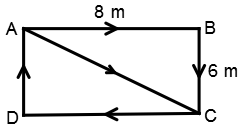# The length and breadth of a room are 8 m and 6 m respectively. A cat runs along all the four walls and finally along a diagonal order to catch a rat. How much total distance is covered by the cat?

## The length and breadth of a room are 8 m and 6 m respectively. A cat runs along all the four walls and finally along a diagonal order to catch a rat. How much total distance is covered by the cat?

A. 10
B. 14
C. 38
D. 48

Solution:Required Distance
= 8 + 6 + 8 + 6 + √(82 + 62)
= 28 + √100
= 28 + 10
= 38 m